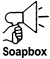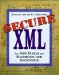# 18.8 Symmetric Key Wrap Algorithms

Symmetric key wrap algorithms are shared secret key encryption algorithms designed for encrypting and decrypting symmetric keys and providing an integrity check. They take, as implicit parameters, their keying material and the key to encrypt or decrypt. Their identifiers appear as Algorithm attributes to EncryptionMethod elements that are children of EncryptedKey, which is in turn a child of KeyInfo (see Figure 18-1). The type of key being wrapped is indicated by the Algorithm attribute of the EncryptionMethod child of the parent of the KeyInfo grandparent of the EncryptionMethod specifying the symmetric key wrap algorithm (see Figure 18-2).

#### 18.8.1 CMS Key Checksum

Some key wrap algorithms use the Key Checksum defined in CMS [RFC 2630]. It provides an integrity check value for the key being wrapped. The algorithm follows:

1. Compute the 20-octet SHA-1 hash of the key being wrapped [FIPS 180-1].

2. Use the first 8 octets of this hash as the checksum value.

#### 18.8.2 Triple DES Key Wrap

` Triple DES Key Wrap Identifiers:     http://www.w3.org/2001/04/xmlenc#kw-tripledes `

Standards-conformant XML Encryption applications must support triple DES wrapping of triple DES keys and may optionally support triple DES wrapping of AES or other keys. An example of a Triple DES Key Wrap EncryptionMethod element follows:

` <EncryptionMethod  Algorithm="http://www.w3.org/2001/04/xmlenc#kw-tripledes"/> `

The following algorithm wraps (encrypts) a key (the wrapped key, WK) under a triple DES key-encrypting key (KEK):

1. Represent the key being wrapped as a byte sequence. If it is a triple DES key, this byte sequence is 24 octets (192 bits) produced by inserting an odd parity bit as the bottom bit of each octet.

2. Compute the key checksum defined in Section 19.12.1; call it CKS.

3. Let WKCKS = WK || CKS, where || means concatenation.

4. Generate eight random octets; call it IV [RFC 1750].

5. Encrypt WKCKS in CBC mode using KEK as the key and IV as the initialization vector. Call the result TEMP1.

6. Let TEMP2 = IV || TEMP1.

7. Reverse the order of the octets in TEMP2; call the result TEMP3.

8. Encrypt TEMP3 in CBC mode using the KEK and an initialization vector of 0x4ADDA22C79E82105. The resulting cipher text is the desired result. It is 40 octets long if a triple DES key is being wrapped.

The following algorithm unwraps (decrypts) a key:

1. Check whether the length of the cipher text is reasonable given the key type. It must be 40 bytes for a triple DES key and either 32, 40, or 48 bytes for an AES key. If the length is wrong, return an error.

2. Decrypt the cipher text with triple DES in CBC mode using the KEK and an IV of 0x4ADDA22C79E82105. Call the output TEMP3.

3. Reverse the order of the octets in TEMP3; call the result TEMP2.

4. Decompose TEMP2 into IV, the first eight octets, and TEMP1, the remaining bytes.

5. Decrypt TEMP1 using triple DES in CBC mode using the KEK and the IV found in Step 4. Call the result WKCKS.

6. Decompose WKCKS. CKS is the last eight octets and WK, the wrapped key, consists of those octets before the CKS.

7. Calculate a CMS Key Checksum over the WK, and compare it with the CKS extracted in Step 6. If they are not equal, return an error.

8. WK is the wrapped key, now extracted for use in data decryption.

This specification matches that given in [RFC 2630].

#### 18.8.3 AES Key Wrap

` AES Key Wrap Identifiers:     http://www.w3.org/2001/04/xmlenc#kw-aes128     http://www.w3.org/2001/04/xmlenc#kw-aes192     http://www.w3.org/2001/04/xmlenc#kw-aes256 `

Implementation of AES key wrap is mandatory under XML Encryption only for 128- and 256-bit key-encrypting keys. None of the versions of AES key wrap takes any explicit parameters; all of them take the usual implicit parameters of encryption algorithms with no initialization vector.

The AES key wrap algorithm described below, as suggested by NIST [AES, AES KMS], provides for confidentiality and integrity. It is defined only for parameters that are multiples of 64 bits. The information wrapped need not actually be a key. The algorithm is the same whatever the size of the AES key used in wrapping, called the key-encrypting key (KEK). Table 18-1 shows the detailed implementation requirements for various key-encrypting key sizes and wrapped data sizes.

##### Table 18-1. AES Key Wrap Implementation Requirements
Key-Encrypting Key Size Wrapped Key Size Requirement
128 128 Required
128 Other than 128 Optional
192 Any Optional
256 256 Required
256 Other than 256 Optional

##### AES Key Wrap Algorithm

Assume that the data to be wrapped consist of N 64-bit data blocks.

If N is 1, then 64 bits are appended, consisting of the repeated octet xA6, and a single AES operation is performed for wrap. Unwrap is also a single AES operation, followed by checking the 64-bit integrity constant and extracting the initial 64 bits of the wrapped quantity.

If N > 1, then 6 * N AES operations are performed for wrap or unwrap. Definitions used in the pseudocode given below are as follows:

• The key to be wrapped is denoted as P(1), P(2), P(3), …, P(N).

• The result of wrapping will be N + 1 64-bit blocks denoted as C(0), C(1), C(2), …, C(N).

• K represents the key-encrypting key.

• Assume integer variables i, j, and t, an intermediate 64-bit register A, a 128-bit register B, and an array of 64-bit quantities R(1) through R(N).

• "|" represents concatenation, so "x | y", where x and y are 64-bit quantities, is the 128-bit quantity with x in the most significant bit positions and y in the least significant bit positions.

• "AES(K)enc(x)" is the operation of AES encrypting the 128-bit quantity x under the key K.

• "AES(K)dec(x)" is the corresponding decryption operation.

• "XOR(x, y)" is the bitwise exclusive OR of x and y.

• "MSB(x)" and "LSB(y)" are the most significant 64 bits and least significant 64 bits of x and y, respectively.One of NIST's explicit design criteria for key wrapping was that every cipher text bit had to be a complex function of every plain text bit after encryption and every plain text bit had to be a complex function of every cipher text bit after decryption. As this is true of single-block AES encryption and decryption, it is obviously true for N = 1. For larger N, the wrap algorithm uses AES to combine adjacent 64-bit blocks so that each of the six iterations of the main loop spread data from a block farther along the data array (wrapping around when it reaches the end). Because the largest AES key is 256 bits (i.e., four 64-bit blocks), you might guess that the main loop count of 6 was chosen so that those four blocks and the one block of integrity check code would be thoroughly mixed. With a fixed count, however, this complete mixing may not occur for larger N. It would seem there is wasted computation for smaller N in the range 1 < N < 4.

##### Wrap

The key wrap algorithm is as follows:

1. If N is 1:

B = AES(K)enc(0xA6A6A6A6A6A6A6A6 | P(1))

C(0) = MSB(B)

C(1) = LSB(B)

If N > 1, perform the following steps:

2. Initialize variables:

Set A to 0xA6A6A6A6A6A6A6A6

For i = 1 to N,

R(i) = P(i)

3. Calculate intermediate values:

For j = 0 to 5,

For i = 1 to N,

t =i + j * N

B = AES(K)enc(A | R(i))

A = XOR(t, MSB(B))

R(i) = LSB(B)

4. Output the results:

Set C(0) = A

For i = 1 to N,

C(i) = R(i)

##### Unwrap

The key unwrap algorithm is as follows:

1. If N is 1:

B = AES(K)dec(C(0) | C(1))

P(1) = LSB(B)

If MSB(B) is 0xA6A6A6A6A6A6A6A6, return success. Otherwise, return an integrity check failure error.

If N > 1, perform the following steps:

2. Initialize the variables:

A = C(0)

For i = 1 to N,

R(i) = C(i)

3. Calculate intermediate values:

For j = 5 to 0,

For i = N to 1,

t =i + j * N

B = AES(K)dec(XOR(t, A) | R(i))

A = MSB(B)

R(i) = LSB(B)

4. Output the results:

For i = 1 to N, P(i) = R(i)

If A is 0xA6A6A6A6A6A6A6A6, return success. Otherwise, return an integrity check failure error.

##### Example

For example, wrapping the data

` 0x00112233445566778899AABBCCDDEEFF `

with the KEK

` 0x000102030405060708090A0B0C0D0E0F `

produces the cipher text of

` 0x1FA68B0A8112B447, 0xAEF34BD8FB5A7B82, 0x9D3E862371D2CFE5 `Secure XML: The New Syntax for Signatures and Encryption
ISBN: 0201756056
EAN: 2147483647
Year: 2005
Pages: 186